# Continuous functions, space of

A normed space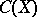of bounded continuous functionson a topological spacewith the norm. Convergence of a sequence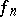inmeans uniform convergence. The spaceis a commutative Banach algebra with a unit element. Ifis compact, then every continuous functionis bounded, consequently,is the space of all continuous functions on.
Whenis a closed interval of real numbers,is denoted by. According to the Weierstrass theorem on the approximation of continuous functions, the set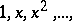of all non-negative integral powers forms a complete system in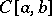. (This means that the set of linear combinations of these powers, that is, polynomials, is everywhere-dense in.) Consequently,is separable; it also has a basis, for example, the Faber–Schauder system of functions forms a basis in. A criterion for compactness inis given by the corresponding theorem of Arzelá: For a certain family of functions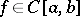to be relatively compact init is necessary and sufficient that the family is uniformly bounded and equicontinuous. This theorem generalizes to the case of the metric space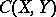of continuous mappings from one metric compactumto another. For the compactness of a closed subsetofit is necessary and sufficient that the mappings inare equicontinuous. The distance between two mappingsandinis given by